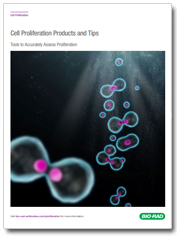# Protocol: alamarBlue Spectrophotometry Calculations with Different Filters

s

### Cell Proliferation Products and TipsTools to Accurately Assess Proliferation

## Overview

1. Make up alamarBlue (AB) as directed in the package insert (1/10 dilution in 100 μI media).
2. Measure the absorbance of alamarBlue in media at the lower wavelength (LW) filter and at the higher wavelength (HW) filter.
3. Measure the absorbance of 100 μl media only (blank) at the two wavelengths.
4. Subtract the absorbance values of media only from the absorbance values of alamarBlue in media.

Where:
AOLW = absorbance of oxidized form at the lower wavelength

AOHW = absorbance of oxidized form at the higher wavelength

AO LW    = absorbance of AB in media  -  absorbance of media only

AO HW  = absorbance of AB in media  -  absorbance of media only

1. Calculate correction factor (Ro):

Correction factor for different filters:

R O  =  AO LW / AO HW

1. Calculate percentage difference in reduction between treated and control cells in cytotoxicity/proliferation assays:

Percentage difference in reduction (equation 5)

=

A LW - ( A HW  R O ) for test well

A LW  - ( A HW  R O ) for control well

x100

Where:
ALW = absorbance at lower wavelength minus the media blank

AHW = absorbance at higher wavelength minus the media blank

Ro = correction factor (calculated in step 5)

1. If the alternative calculation to find the percentage reduction of alamarBlue is required, use the following equation:

 Percentage reduction of alamarBlue (equation 6) =    [  A LW  - ( A HW  x  R O ) ]  x 100

### Example Data:

E. coli was added to Mueller Hinton broth and alamarBlue to a final concentration of 1 x 106 cfu/ml. The broth was incubated at 36oC and readings taken of 1 ml samples every 30 min, absorbance was measured at 560 nm and 605 nm.

Table 1. Example data (test wells contain 10% alamarBlue  in 100 μl​ media containing cells).

Absorbance 560 nm Absorbance 605 nm
10% alamarBlue in 100 μl media 0.474 0.815
100 μl media 0.082 0.037
Test well after 60 min incubation 0.639 0.889
Test well after 120 min incubation 0.797 0.893

Where AO LW   = 0.474 - 0.082 = 0.392

Where AO HW = 0.815 - 0.037 = 0.778

R O  = 0.392 / 0.778 = 0.504     [Using equation 6]

Where:

A LW =absorbance at lower wavelength minus the media blank

A HW =absorbance at higher wavelength minus the media blank

R O = Correction factor (calculated in previous step)

After 60 min incubation:                  After 120 min incubation:
ALW = 0.639 - 0.082 = 0.557   ALW
AHW = 0.889 - 0.037 = 0.852   AHW
R O = 0.504   R O
Percentage reduction of alamarBlue   Percentage reduction of alamarBlue
= [0.557 - (0.852 x 0.504)] x 100    = [0.715 - (0.856 x 0.504)] x 100
= 13%   =28%

Related products